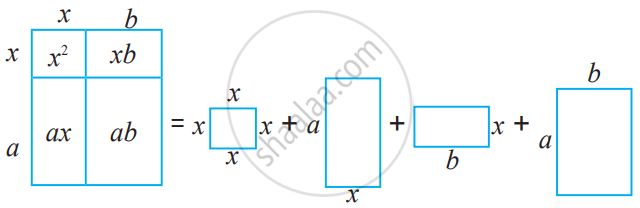# Expansion of (x + a)(x + b)

#### formula

• (x + a)(x + b) = x2 + (a + b)x + ab

# Expansion of (x + a)(x + b):

Expand (x + a)(x + b) using formulae for areas of a square and a rectangle.(x + a) and (x + b) are binomials with one term in common. Let us multiply them.

(x + a)(x + b) = x(x + b) +a(x + b)

(x + a)(x + b) = x2 + bx + ax + ab

(x + a)(x + b) = x2 + (a + b)x + ab.

#### Example

Use the Identity (x + a) (x + b) = x2 + (a + b)x + ab to find the following:

95 × 103

95 × 103
= (100 - 5) × (100 + 3)
= 1002 + (-5 + 3) × 100 + (-5) × 3
= 10000 - 200 - 15
= 9785.

#### Example

Use the Identity (x + a)(x + b) = x2 + (a + b)x + ab to find the following:
501 × 502

501 × 502
= (500 + 1) × (500 + 2)
= 5002 + (1 + 2) × 500 + 1 × 2
= 250000 + 1500 + 2
= 251502.

#### Example

Expand: (y + 4)(y - 3)

(y + 4)(y - 3)

= y2 + (4 - 3)y + (4) × (-3)

= y2 + y - 12.

#### Example

Expand: (2a + 3b)(2a - 3b).

(2a + 3b)(2a - 3b)

= (2a)2 + [(3b) + (- 3b)]2a + [3b × (-3b)]

= 4a2 + 0  × 2a - 9b2

= 4a2 - 9b2

#### Example

Expand: (m + 3/2)(m + 1/2).

(m + 3/2)(m + 1/2).

= m^2 + (3/2 + 1/2)m + 3/2 xx 1/2

= m^2 + 2m + 3/4.

#### Example

Expand: (x - 3)(x - 7).

(x - 3)(x - 7)

= x2 + (-3 - 7)x + (-3)(-7)

= x2 - 10x + 21.

#### Example

Expand: (x + 2)(x + 3).

(x + 2)(x + 3)

= x2 + (2 + 3)x + (2 × 3)

= x2 + 5x + 6.

If you would like to contribute notes or other learning material, please submit them using the button below.

### Shaalaa.com

Expansion of (x + a)(x + b) [00:02:23]
S
0%